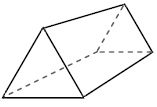# Classifying Solids Online Quiz

Following quiz provides Multiple Choice Questions (MCQs) related to Classifying Solids. You will have to read all the given answers and click over the correct answer. If you are not sure about the answer then you can check the answer using Show Answer button. You can use Next Quiz button to check new set of questions in the quiz.Q 1 - Use the diagram to identify the name of the following shape.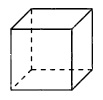### Explanation

Step 1:

The given figure is a prism whose length, width and height are equal in length.

Step 2:

The given figure is a cube

Q 2 - Use the diagram to identify the name of the following shape.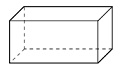### Explanation

Step 1:

The given figure is a prism whose base is a rectangle.

Step 2:

The given figure is a rectangular prism.

Q 3 - Use the diagram to identify the name of the following shape.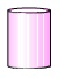### Explanation

Step 1:

The given figure is a prism whose base is a circle.

Step 2:

The given figure is a cylinder

Q 4 - Use the diagram to identify the name of the following shape.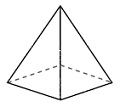### Explanation

Step 1:

The given figure is a pyramid whose base is a square.

Step 2:

The given figure is a square pyramid.

Q 5 - Use the diagram to identify the name of the following shape.### Explanation

Step 1:

The given figure is a prism whose base is a rectangle.

Step 2:

The given figure is a rectangular prism.

Q 6 - Use the diagram to identify the name of the following shape.### Explanation

Step 1:

The given figure is a three dimensional round ball like shape.

Step 2:

The given figure is a sphere.

Q 7 - Use the diagram to identify the name of the following shape.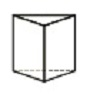### Explanation

Step 1:

The given figure is a prism whose base is a triangle.

Step 2:

The given figure is a triangular prism.

Q 8 - Use the diagram to identify the name of the following shape.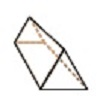### Explanation

Step 1:

The given figure is a prism whose base is a triangle.

Step 2:

The given figure is a triangular prism.

Q 9 - Use the diagram to identify the name of the following shape.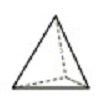### Explanation

Step 1:

The given figure is a pyramid whose base is a triangle.

Step 2:

The given figure is a triangular pyramid.

Q 10 - Use the diagram to identify the name of the following shape.